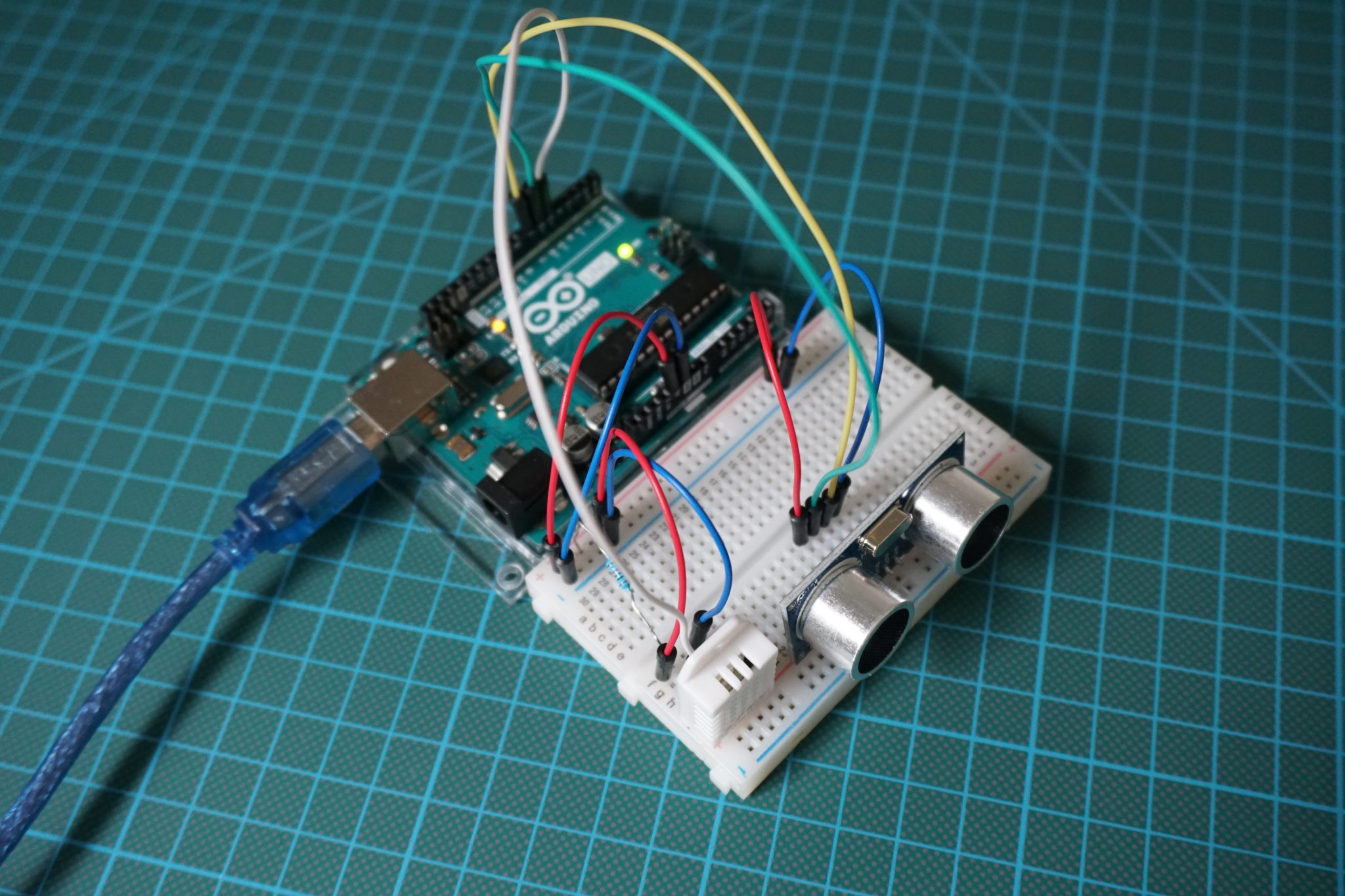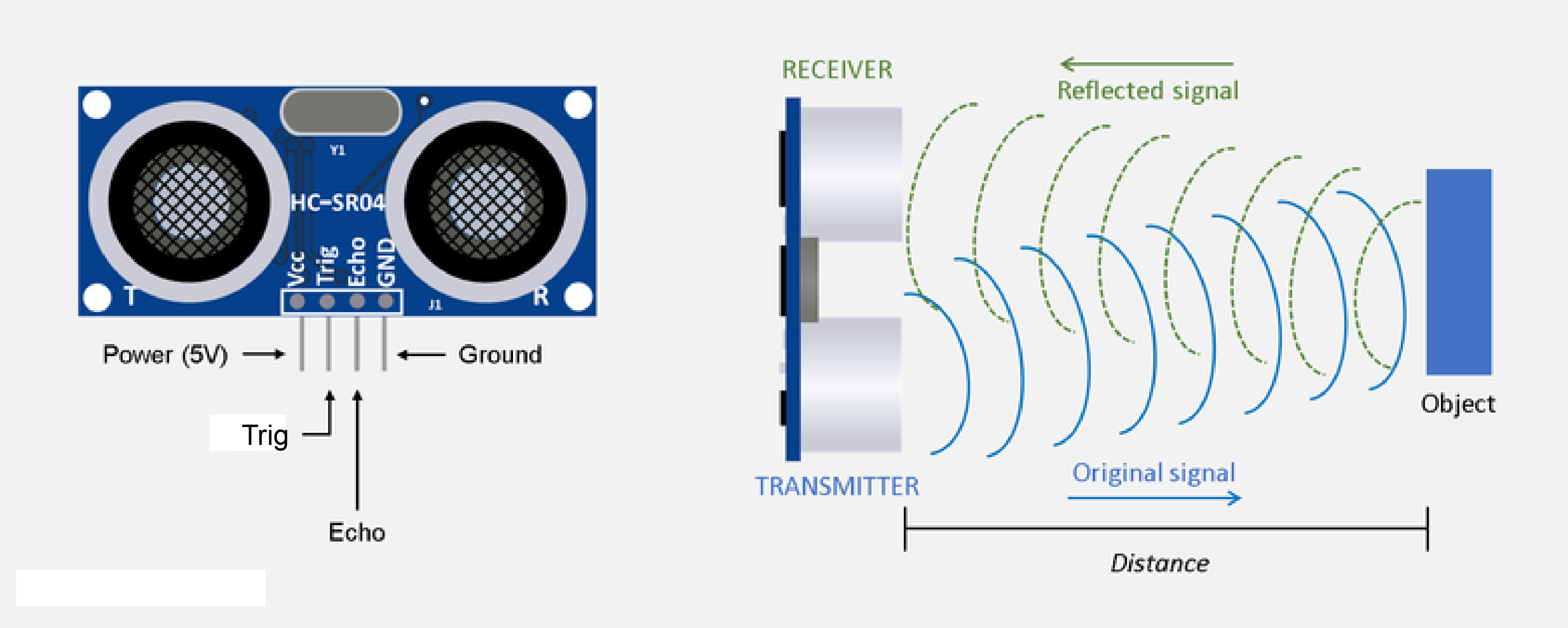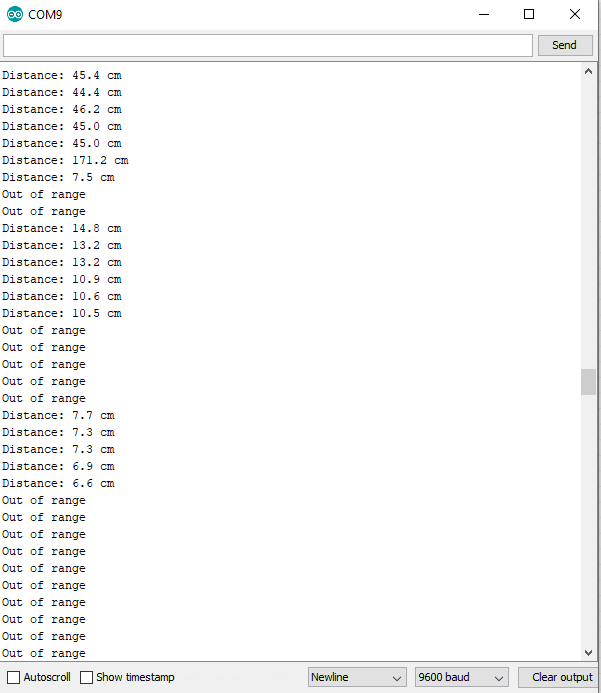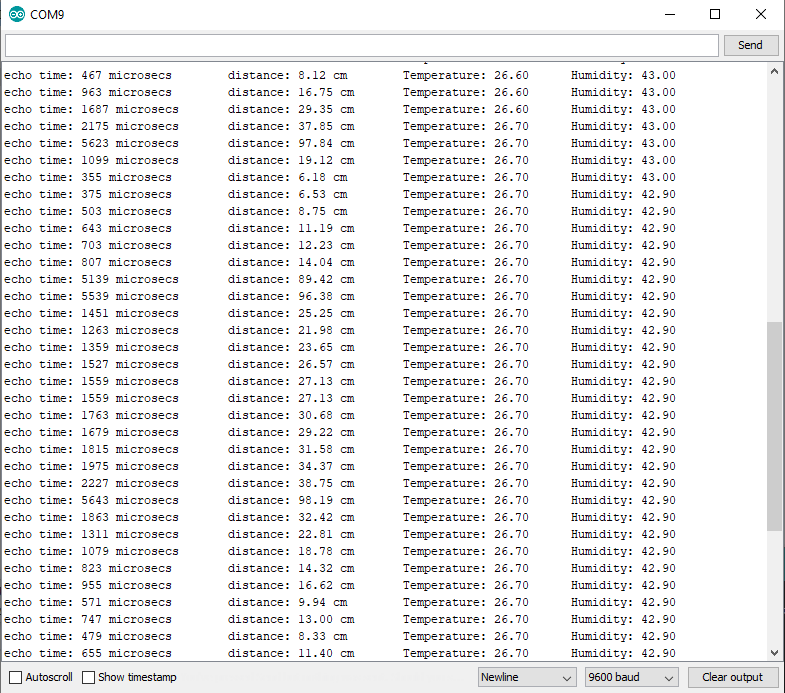# Ultrasonic Sensor Tutorial for Arduino, ESP8266 and ESP32

In this tutorial you learn how to use the ultrasonic distance sensor HC-SR04 to measure the distance between the sensor and an object.

You learn how ultrasonic works in general, the wiring between the HC-SR04 module and different Arduino, ESP8266 and ESP32 microcontroller boards and how to program the code to measure the distance.

In the last example we improve the measurement by including the temperature and humidity to the calculation.## Functionality of an Ultrasonic Distance Sensor

The ultrasonic distance sensor is used to measure the distance between the sensor and an object. There are a lot of robotic projects which use the sensor to identify a wall in a labyrinth and rotate the robot with the objective to find a way out of the labyrinth.

The most used sensor is the HC-SR04 ultrasonic distance sensor that is shown in the following picture.This sensor can measure a distances between 2cm and 4m. The measuring is done by the time taken to receive the echo of a 40 kHz ultrasonic sound wave that is emitted by the sensor, reflected by the object and received by the receiver of the ultrasonic distance sensor.

For reliable measurements the ultrasonic distance sensor should be perpendicular to the scanned surface in horizontal and vertical direction. To further improve the accuracy of the measurement, the scanned surface should be flat.

### HC-SR04 Ultrasonic Distance Sensor Datasheet

The HC-SR04 ultrasonic distance sensor has the following technical specifications:

 Power Supply 1. Gen 4.8V...5V DC 2. Gen 3V...5V DC Quiescent Current <2mA Working Current 15mA Effectual Angle <15° Ranging Distance 2cm...400cm 1″...13ft Resolution 0.3 cm Measuring Angle 30° Trigger Input Pulse width 10uS Dimension 45mm x 20mm x 15mm

The following table gives you an overview of all components and parts that I used for this tutorial. I get commissions for purchases made through links in this table.

Arduino Nano AmazonAliExpress
Arduino Pro Mini AmazonAliExpress
Arduino Uno AmazonAliExpress
Arduino Mega AmazonAliExpress
ESP32 ESP-WROOM-32AmazonAliExpress
ESP8266 NodeMCU AmazonAliExpress
ESP8266 WeMos D1 Mini AmazonAliExpress
HC-SR04 Ultrasonic Distance Sensor and Temperature Sensor in Sensor Kit AmazonAliExpress

## Basic Distance Measurement with Ultrasonic Sensor

The following example will give you a quick overview of the equations how to measure the distance with an ultrasonic distance sensor. The sensor send an eight-cycle signal at 40 kHz. After time_1 the signal will be reflected by the object and after time_2 the signal return back to the sensor and is measured. Therefore the distance between the sensor and the object is the speed of the sound multiplied by half of the sum of the time the signal was send, reflected and received.

With the total time, the the ultrasonic signal needed to get back to the sensor and the knowledge that the speed of sound is 343 m/s, we can calculate the distance between the ultrasonic sensor module and the object. Hint: We will use a library that does this job for us 🙂

### Wiring between HC-SR04 Ultrasonic Distance Sensor and Microcontroller

If you have the first generation of the HC-SR04 and use the ESP8266 or ESP32 you have to power the ultrasonic distance sensor from the 5V power supply of the USB connection (VIN, VV, V5). But if you have the second generation, you can also use the standard 3.3V output of the microcontroller.

The following pictures show the wiring between the HC-SR04 Ultrasonic Distance Sensor and different Arduino, ESP8266 and ESP32 microcontroller.Microcontroller Datasheet eBook

The 35 pages Microcontroller Datasheet Playbook contains the most useful information of 14 Arduino, ESP8266 and ESP32 microcontroller boards.

### Code to Measure the Distance with the HC-SR04 Module

After we connect our devices we will go into the code. Our objective is to measure the distance between the sensor and our hand and print the distance to the serial monitor output. If the distance is out of range (< 2cm or > 4m) we will print an error.

For the implementation we use the library for the HC-SR04 ultrasonic distance sensor called NewPing library by Tim Eckel. If you do not know how to install a new library in your Arduino IDE, I wrote a step by step tutorial how to install libraries.

The NewPing library has some very nice functions. Here are the top 3 of them:

• sonar.ping_cm(): Returns the distance between the sensor and the target, but there are no digits after
the decimal point.
• sonar.convert_cm(echotime): Returns the distance given the echo time, but outlier values can be observed.
It is more robust to calculate the distance between the sensor and point directly from the echo time.
• sonar.ping_median(number of observations): Returns median echo time for the number of observations, with a minimum of five observations, after excluding out-of-range values.
```#include "NewPing.h"      // include NewPing library

// for Arduino microcontroller
int trigPin = 6;      // trigger pin
int echoPin = 7;      // echo pin

// for ESP8266 microcontroller
//int trigPin = D0;      // trigger pin
//int echoPin = D1;      // echo pin

// for ESP32 microcontroller
//int trigPin = 4;      // trigger pin
//int echoPin = 0;      // echo pin

NewPing sonar(trigPin, echoPin);

void setup(){
Serial.begin(9600);
}

void loop(){
float distance = sonar.ping_median(5);

if(distance>400 || distance<2) Serial.println("Out of range");
else
{
Serial.print("Distance: ");
Serial.print(distance, 1); Serial.println(" cm");
}

delay(50);
}```

The first set is to include the NewPing library that we want to use. Then we have to define the connection pins between our microcontroller and the HC-SR04 ultrasonic distance sensor. Because I created this script for Arduino, ESP8266 and ESP32 microcontroller, you only have to use one section of the following three that is created for your microcontroller. You can either delete or comment the sections that you do not need.

After we include the library and define the connection pins we create a NewPing object, called sonar, that has two arguments: the trigger and the echo pin.

In the setup function, we set the baud rate to 9600 that has to match the baud rate of your serial monitor or serial plotter in the Arduino IDE.

In the loop function, we use the ping_median function of our created sonar object to get the distance of the detected object in cm and save the distance to a new variable. You can also use the ping_cm function at this point.

Regarding our objective in example, if the distance is greater than 4m or shorter than 2cm, we want to print in the serial monitor, that the object is out of range. Otherwise we want to print the distance to the serial monitor. This is done in the program script via a if-else statement.

At the end of the loop function we wait 50ms and start the loop function all over again.

The following picture shows the serial monitor of the Arduino IDE with the measured distance and you see also the message when the distance is out of range.## Improved Distance Measurement with Ultrasonic Sensor with Temperature and Humidity

In the first example the distance is calculated with a speed of sound of 343 m/s that gives us a good accuracy of the distance. But the speed of sound depends on the temperature and humidity where the ultrasonic wave moves. Therefore we can improve the accuracy using the following equation that calculates the speed of sound in dependence of the temperature and the humidity: v_sound = 331.3 + (0.606 * temp°C) + (0.0124 * humidity) m/s.

The following example we will use different Arduino, ESP8266 and ESP32 microcontroller and also a temperature and humidity sensor to optimize the distance measurement.

### Wiring between HC-SR04, DHT22 and Microcontroller

If you have the first generation of the HC-SR04 and use the ESP8266 or ESP32 you have to power the ultrasonic distance sensor from the 5V power supply of the USB connection (VIN, VV, V5). But if you have the second generation, you can also use the standard 3.3V output of the microcontroller.

The following pictures show the wiring between the HC-SR04 Ultrasonic Distance Sensor, the DHT22 temperature and humidity sensor and different Arduino, ESP8266 and ESP32 microcontroller.

If you want to know more about temperature and humidity sensors, there is a whole article about different temperature and humidity sensors, including the DHT11 and DHT22.

### Code to Improve the Distance Measurement with the HC-SR04 Module

In the program code we added the reading of the temperature sensor and we calculated the speed of sound like the equation. Also we added some variables in the serial output:

• echoTime: Raw value from the HC-SR04 ultrasonic distance sensor to calculate the distance between the sensor and the object.
• distance: The distance between the sensor and the object.
• temperature and humidity: The temperature and humidity from the DHT22 to calculate the speed of sound.
```#include "NewPing.h"      // include NewPing library
#include "DHT.h"
#define DHT22TYPE DHT22   // DHT22 type

// for Arduino microcontroller
int DHT22PIN = 5;     // DHT22 pin
int trigPin = 6;      // trigger pin
int echoPin = 7;      // echo pin

// for ESP8266 microcontroller
//int DHT22PIN = D2;     // DHT22 pin
//int trigPin = D0;      // trigger pin
//int echoPin = D1;      // echo pin

// for ESP32 microcontroller
//int DHT22PIN = 2;     // DHT22 pin
//int trigPin = 4;      // trigger pin
//int echoPin = 0;      // echo pin

NewPing sonar(trigPin, echoPin);
DHT dht22(DHT22PIN, DHT22TYPE);

void setup(){
Serial.begin(9600);
dht22.begin();
}

void loop(){
int echoTime = sonar.ping();          // echo time (μs)
float vsound = 331.3+(0.606*temperature)+(0.0124*humidity);
float distance = (echoTime/2.0)*vsound/10000; // distance between sensor and target in cm

Serial.print("echo time: ");
Serial.print(echoTime);
Serial.print(" microsecs\t");
Serial.print("distance: ");
Serial.print(distance,2);
Serial.print(" cm");
Serial.print("\t Temperature: ");
Serial.print(temperature);
Serial.print("\t Humidity: ");
Serial.println(humidity);
delay(50);
}```

Most of the Arduino program code is the same compared to the first example. Therefore I will only concentrate on the lines that are different.

In the first part of the program code, we have to add the DHT library for the temperature and humidity sensor. Because there are different DHT modules, we have to define that our used DHT sensor is a DHT22 sensor. If you use a DHT11, you have to change this line.

Next we have to add the pin that connects the DHT sensor to the microcontroller. In each of the three sections we add the connection pin that we get from the previous wiring section.

Like the ultrasonic sensor, we have to create a new DHT object called dht22 that gets the connection pin of the DHT sensor and the DHT type.

In the setup function we have to initialize the dht22 object with dht22.begin().

The magic happens in the loop function. Instead of receiving the distance from the NewPing library, we only get the echo time in μs. Next we get the temperature and the humidity from the DHT sensor and calculate the speed of sound with the equation.

Now the distance between the HC-SR04 and the object is half the echo time (HC-SR04 -> object -> HC-SR04) multiplied with the speed of sound and divided by 10,000 to get the distance in cm.

At the end we print the echo time, the distance, temperature and humidity to the serial monitor of the Arduino IDE that you see in the following picture.Do you have any further questions about the ultrasonic distance sensor? Use the comment section below to ask your questions. And what are projects you would like to use the distance sensor? There is also a second sensor to detect objects in a predefined range, the infrared distance module.

### 1 thought on “Ultrasonic Sensor Tutorial for Arduino, ESP8266 and ESP32”

1. Merci pour le partage claire net et précis.